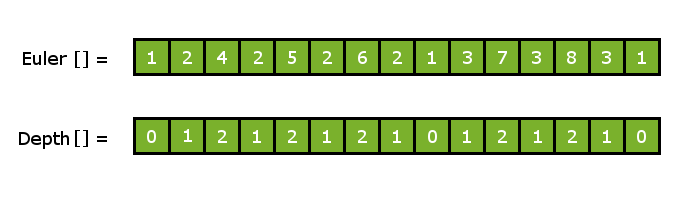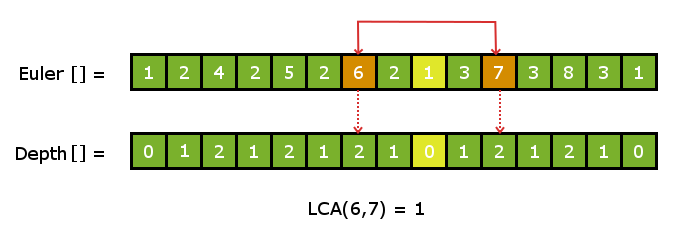# LCA for n-ary Tree | Constant Query O(1)

We have seen various methods with different Time Complexities to calculate LCA in n-ary tree:-
Method 1 : Naive Method ( by calculating root to node path) | O(n) per query
Method 2 :Using Sqrt Decomposition | O(sqrt H)
Method 3 : Using Sparse Matrix DP approach | O(logn)

Lets study another method which has faster query time than all the above methods. So, our aim will be to calculate LCA in constant time ~ O(1). Let’s see how we can achieve it.

Method 4 : Using Range Minimum Query

We have discussed LCA and RMQ for binary tree. Here we discuss LCA problem to RMQ problem conversion for n-ary tree.

```Pre-requisites:- LCA in Binary Tree using RMQ
RMQ using sparse table
```

Key Concept : In this method, we will be reducing our LCA problem to RMQ(Range Minimum Query) problem over a static array. Once, we do that then we will relate the Range minimum queries to the required LCA queries.

The first step will be to decompose the tree into a flat linear array. To do this we can apply the Euler walk . The Euler walk will give the pre-order traversal of the graph. So we will perform a Euler Walk on the tree and store the nodes in an array as we visit them. This process reduces the tree data-structure to a simple linear array.

Consider the below tree and the euler walk over it :-Now lets think in general terms : Consider any two nodes on the tree. There will be exactly one path connecting both the nodes and the node that has the smallest depth value in the path will be the LCA of the two given nodes.
Now take any two distinct node say u and v in the Euler walk array. Now all the elements in the path from u to v will lie in between the index of nodes u and v in the Euler walk array. Therefore, we just need to calculate the node with the minimum depth between the index of node u and node v in the euler array.

For this we will maintain another array that will contain the depth of all the nodes corresponding to their position in the Euler walk array so that we can Apply our RMQ algorithm on it.

Given below is the euler walk array parallel to its depth track array.Example :- Consider two nodes node 6 and node 7 in the euler array. To calculate the LCA of node 6 and node 7 we look the smallest depth value for all the nodes in between node 6 and node 7 .
Therefore, node 1 has the smallest depth value = 0 and hence, it is the LCA for node 6 and node 7.Implementation:-

```We will be maintaining three arrays 1)Euler Path
2)Depth array
3)First Appearance Index
```

Euler Path and Depth array are the same as described above

First Appearance Index FAI[] : The First Appearance index Array will store the index for the first position of every node in the Euler Path array. FAI[i] = First appearance of ith node in Euler Walk array.

The Implementation for the above method is given below:-

## C++

 `// C++ program to demonstrate LCA of n-ary tree` `// in constant time.` `#include "bits/stdc++.h"` `using` `namespace` `std;` `#define sz 101`   `vector < ``int` `> adj[sz];    ``// stores the tree` `vector < ``int` `> euler;      ``// tracks the eulerwalk` `vector < ``int` `> depthArr;   ``// depth for each node corresponding` `                           ``// to eulerwalk`   `int` `FAI[sz];     ``// stores first appearence index of every node` `int` `level[sz];   ``// stores depth for all nodes in the tree` `int` `ptr;         ``// pointer to euler walk` `int` `dp[sz];  ``// sparse table` `int` `logn[sz];    ``// stores log values` `int` `p2;      ``// stores power of 2`   `void` `buildSparseTable(``int` `n)` `{` `    ``// initializing sparse table` `    ``memset``(dp,-1,``sizeof``(dp));`   `    ``// filling base case values` `    ``for` `(``int` `i=1; idepthArr[i-1])?i-1:i;`   `    ``// dp to fill sparse table` `    ``for` `(``int` `l=1; l<15; l++)` `      ``for` `(``int` `i=0; idepthArr[dp[i+p2[l-1]][l-1]])?` `             ``dp[i+p2[l-1]][l-1] : dp[i][l-1];` `        ``else` `             ``break``;` `}`   `int` `query(``int` `l,``int` `r)` `{` `    ``int` `d = r-l;` `    ``int` `dx = logn[d];` `    ``if` `(l==r) ``return` `l;` `    ``if` `(depthArr[dp[l][dx]] > depthArr[dp[r-p2[dx]][dx]])` `        ``return` `dp[r-p2[dx]][dx];` `    ``else` `        ``return` `dp[l][dx];` `}`   `void` `preprocess()` `{` `    ``// memorizing powers of 2` `    ``p2 = 1;` `    ``for` `(``int` `i=1; i<18; i++)` `        ``p2[i] = p2[i-1]*2;`   `    ``// memorizing all log(n) values` `    ``int` `val = 1,ptr=0;` `    ``for` `(``int` `i=1; i FAI[v])` `       ``swap(u,v);`   `    ``// doing RMQ in the required range` `    ``return` `euler[query(FAI[u], FAI[v])];` `}`   `void` `addEdge(``int` `u,``int` `v)` `{` `    ``adj[u].push_back(v);` `    ``adj[v].push_back(u);` `}`   `int` `main(``int` `argc, ``char` `const` `*argv[])` `{` `    ``// constructing the described tree` `    ``int` `numberOfNodes = 8;` `    ``addEdge(1,2);` `    ``addEdge(1,3);` `    ``addEdge(2,4);` `    ``addEdge(2,5);` `    ``addEdge(2,6);` `    ``addEdge(3,7);` `    ``addEdge(3,8);`   `    ``// performing required precalculations` `    ``preprocess();`   `    ``// doing the Euler walk` `    ``ptr = 0;` `    ``memset``(FAI,-1,``sizeof``(FAI));` `    ``dfs(1,0,0);`   `    ``// creating depthArray corresponding to euler[]` `    ``makeArr();`   `    ``// building sparse table` `    ``buildSparseTable(depthArr.size());`   `    ``cout << ``"LCA(6,7) : "` `<< LCA(6,7) << ``"\n"``;` `    ``cout << ``"LCA(6,4) : "` `<< LCA(6,4) << ``"\n"``;`   `    ``return` `0;` `}`

## Java

 `// Java program to demonstrate LCA of n-ary` `// tree in constant time.` `import` `java.util.ArrayList;` `import` `java.util.Arrays;`   `class` `GFG{`   `static` `int` `sz = ``101``;`   `@SuppressWarnings``(``"unchecked"``)` `// Stores the tree` `static` `ArrayList[] adj = ``new` `ArrayList[sz]; `   `// Tracks the eulerwalk` `static` `ArrayList euler = ``new` `ArrayList<>(); `   `// Depth for each node corresponding` `static` `ArrayList depthArr = ``new` `ArrayList<>(); ` `// to eulerwalk`   `// Stores first appearence index of every node` `static` `int``[] FAI = ``new` `int``[sz]; `   `// Stores depth for all nodes in the tree` `static` `int``[] level = ``new` `int``[sz]; `   `// Pointer to euler walk` `static` `int` `ptr;`   `// Sparse table` `static` `int``[][] dp = ``new` `int``[sz][``18``];`   `// Stores log values` `static` `int``[] logn = ``new` `int``[sz];`   `// Stores power of 2` `static` `int``[] p2 = ``new` `int``[``20``];`   `static` `void` `buildSparseTable(``int` `n)` `{` `    `  `    ``// Initializing sparse table` `    ``for``(``int` `i = ``0``; i < sz; i++)` `    ``{` `        ``for``(``int` `j = ``0``; j < ``18``; j++) ` `        ``{` `            ``dp[i][j] = -``1``;` `        ``}` `    ``}`   `    ``// Filling base case values` `    ``for``(``int` `i = ``1``; i < n; i++)` `        ``dp[i - ``1``][``0``] = (depthArr.get(i) > ` `                        ``depthArr.get(i - ``1``)) ? ` `                                     ``i - ``1` `: i;`   `    ``// dp to fill sparse table` `    ``for``(``int` `l = ``1``; l < ``15``; l++)` `        ``for``(``int` `i = ``0``; i < n; i++)` `            ``if` `(dp[i][l - ``1``] != -``1` `&&` `               ``dp[i + p2[l - ``1``]][l - ``1``] != -``1``)` `                ``dp[i][l] = (depthArr.get(dp[i][l - ``1``]) >` `                            ``depthArr.get(` `                                ``dp[i + p2[l - ``1``]][l - ``1``])) ? ` `                                ``dp[i + p2[l - ``1``]][l - ``1``] : ` `                                ``dp[i][l - ``1``];` `            ``else` `                ``break``;` `}`   `static` `int` `query(``int` `l, ``int` `r) ` `{` `    ``int` `d = r - l;` `    ``int` `dx = logn[d];` `    `  `    ``if` `(l == r)` `        ``return` `l;` `        `  `    ``if` `(depthArr.get(dp[l][dx]) > ` `        ``depthArr.get(dp[r - p2[dx]][dx]))` `        ``return` `dp[r - p2[dx]][dx];` `    ``else` `        ``return` `dp[l][dx];` `}`   `static` `void` `preprocess() ` `{` `    `  `    ``// Memorizing powers of 2` `    ``p2[``0``] = ``1``;` `    ``for``(``int` `i = ``1``; i < ``18``; i++)` `        ``p2[i] = p2[i - ``1``] * ``2``;`   `    ``// Memorizing all log(n) values` `    ``int` `val = ``1``, ptr = ``0``;` `    ``for``(``int` `i = ``1``; i < sz; i++) ` `    ``{` `        ``logn[i] = ptr - ``1``;` `        ``if` `(val == i) ` `        ``{` `            ``val *= ``2``;` `            ``logn[i] = ptr;` `            ``ptr++;` `        ``}` `    ``}` `}`   `// Euler Walk ( preorder traversal) converting` `// tree to linear depthArray ` `// Time Complexity : O(n)` `static` `void` `dfs(``int` `cur, ``int` `prev, ``int` `dep)` `{` `    `  `    ``// Marking FAI for cur node` `    ``if` `(FAI[cur] == -``1``)` `        ``FAI[cur] = ptr;`   `    ``level[cur] = dep;`   `    ``// Pushing root to euler walk` `    ``euler.add(cur);`   `    ``// Incrementing euler walk pointer` `    ``ptr++;`   `    ``for``(Integer x : adj[cur])` `    ``{` `        ``if` `(x != prev)` `        ``{` `            ``dfs(x, cur, dep + ``1``);`   `            ``// Pushing cur again in backtrack` `            ``// of euler walk` `            ``euler.add(cur);`   `            ``// Increment euler walk pointer` `            ``ptr++;` `        ``}` `    ``}` `}`   `// Create Level depthArray corresponding` `// to the Euler walk Array` `static` `void` `makeArr()` `{` `    ``for``(Integer x : euler)` `        ``depthArr.add(level[x]);` `}`   `static` `int` `LCA(``int` `u, ``int` `v) ` `{` `    `  `    ``// Trival case` `    ``if` `(u == v)` `        ``return` `u;`   `    ``if` `(FAI[u] > FAI[v])` `    ``{` `        ``int` `temp = u;` `        ``u = v;` `        ``v = temp;` `    ``}`   `    ``// Doing RMQ in the required range` `    ``return` `euler.get(query(FAI[u], FAI[v]));` `}`   `static` `void` `addEdge(``int` `u, ``int` `v)` `{` `    ``adj[u].add(v);` `    ``adj[v].add(u);` `}`   `// Driver code` `public` `static` `void` `main(String[] args)` `{` `    ``for``(``int` `i = ``0``; i < sz; i++)` `    ``{` `        ``adj[i] = ``new` `ArrayList<>();` `    ``}` `    `  `    ``// Constructing the described tree` `    ``int` `numberOfNodes = ``8``;` `    ``addEdge(``1``, ``2``);` `    ``addEdge(``1``, ``3``);` `    ``addEdge(``2``, ``4``);` `    ``addEdge(``2``, ``5``);` `    ``addEdge(``2``, ``6``);` `    ``addEdge(``3``, ``7``);` `    ``addEdge(``3``, ``8``);`   `    ``// Performing required precalculations` `    ``preprocess();`   `    ``// Doing the Euler walk` `    ``ptr = ``0``;` `    ``Arrays.fill(FAI, -``1``);` `    ``dfs(``1``, ``0``, ``0``);`   `    ``// Creating depthArray corresponding to euler[]` `    ``makeArr();` `    `  `    ``// Building sparse table` `    ``buildSparseTable(depthArr.size());`   `    ``System.out.println(``"LCA(6,7) : "` `+ LCA(``6``, ``7``));` `    ``System.out.println(``"LCA(6,4) : "` `+ LCA(``6``, ``4``));` `}` `}`   `// This code is contributed by sanjeev2552`

Output:

```LCA(6,7) : 1
LCA(6,4) : 2
```

Note : We are precalculating all the required power of 2’s and also precalculating the all the required log values to ensure constant time complexity per query. Else if we did log calculation for every query operation our Time complexity would have not been constant.

Time Complexity: The Conversion process from LCA to RMQ is done by Euler Walk that takes O(n) time.
Pre-processing for the sparse table in RMQ takes O(nlogn) time and answering each Query is a Constant time process. Therefore, overall Time Complexity is O(nlogn) – preprocessing and O(1) for each query.

This article is contributed by Nitish Kumar. If you like GeeksforGeeks and would like to contribute, you can also write an article using contribute.geeksforgeeks.org or mail your article to contribute@geeksforgeeks.org. See your article appearing on the GeeksforGeeks main page and help other Geeks.

Attention reader! Don’t stop learning now. Get hold of all the important DSA concepts with the DSA Self Paced Course at a student-friendly price and become industry ready.

My Personal Notes arrow_drop_up

Improved By : Akanksha_Rai, sanjeev2552

Article Tags :
Practice Tags :

5

Please write to us at contribute@geeksforgeeks.org to report any issue with the above content.